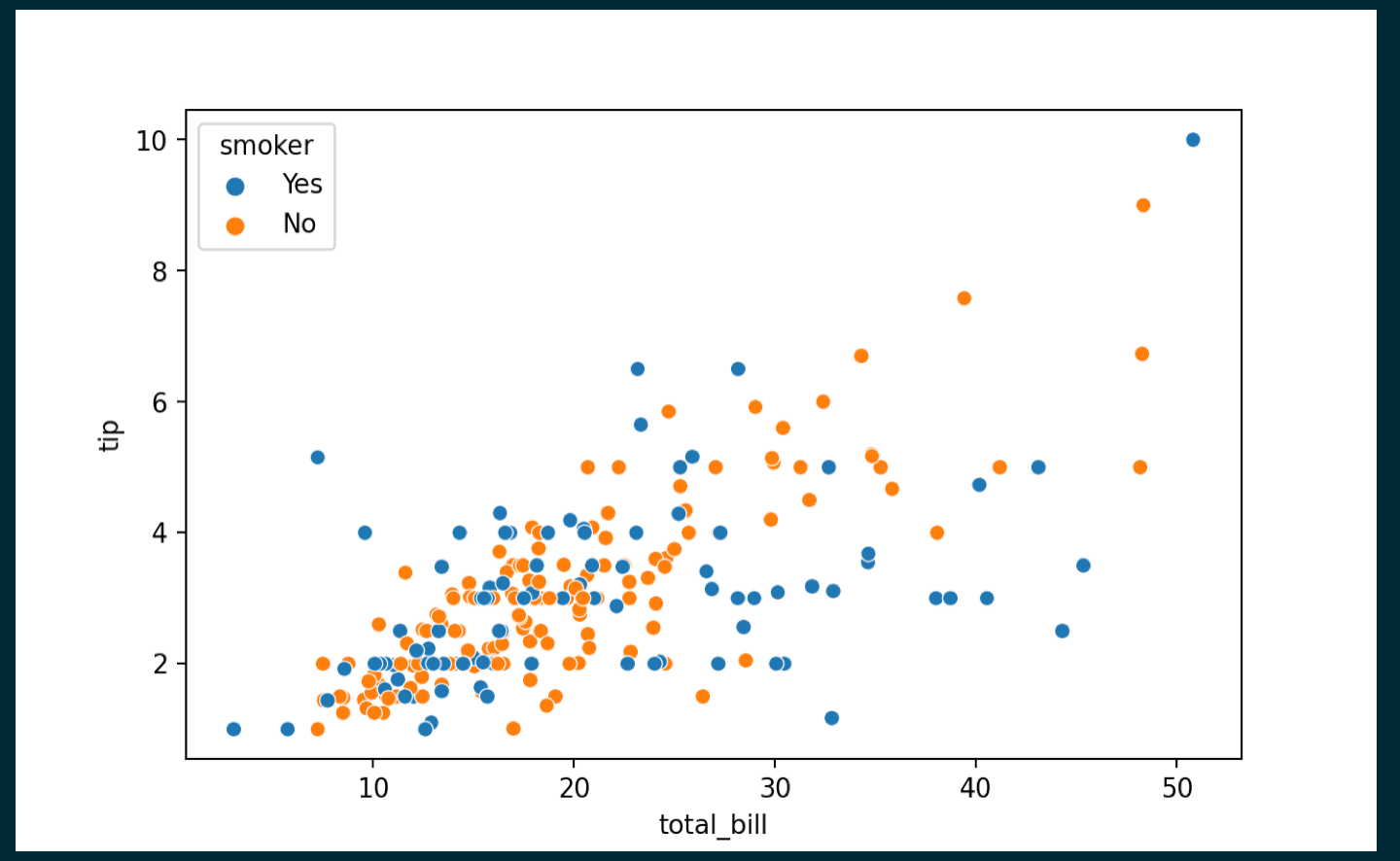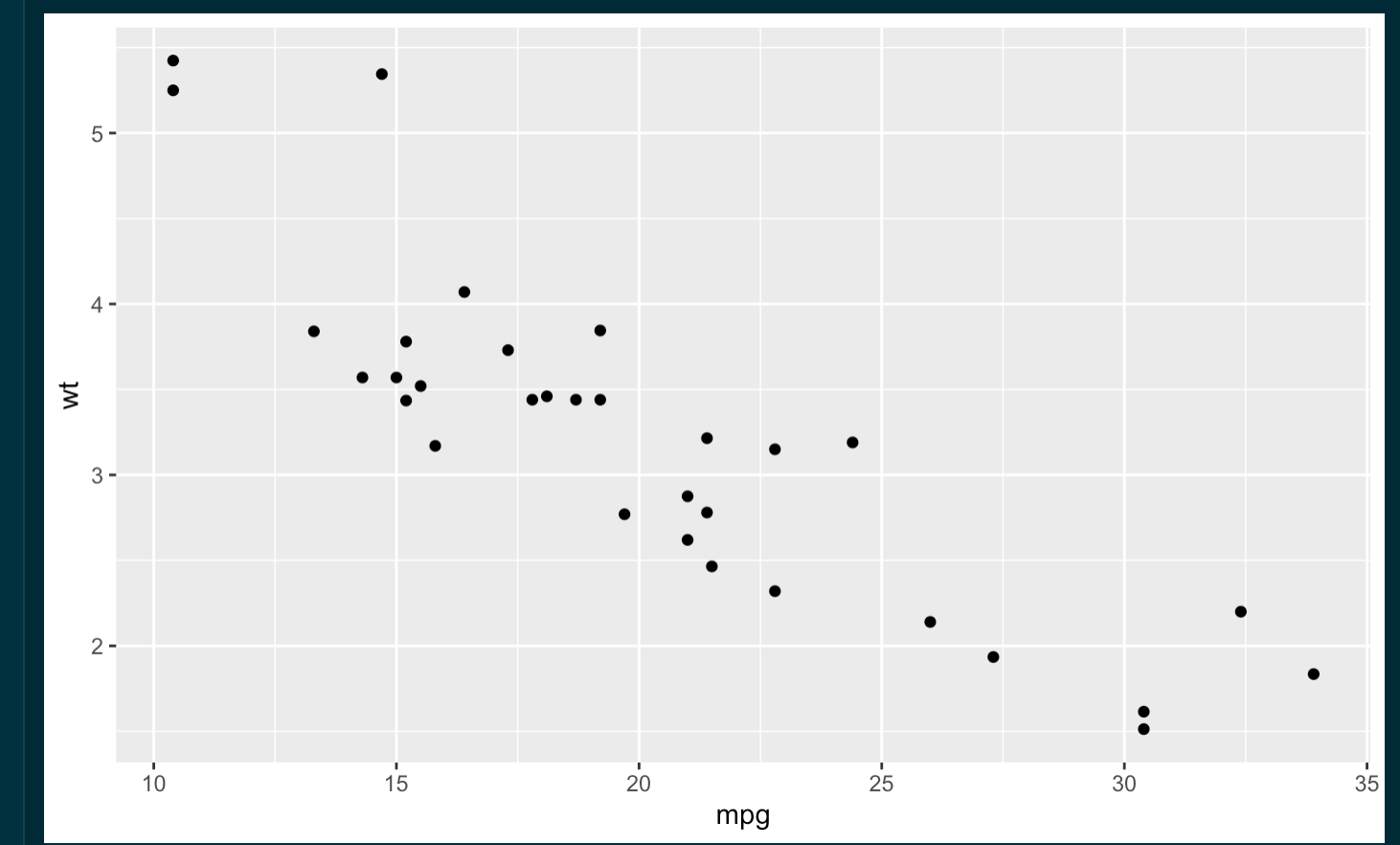WHCSRL 技术网

# R markdown中使用reticulate_qq

``````output:
prettydoc::html_pretty:
theme: architect
highlight: github
```1234```
``````library(reticulate)
use_condaenv("BERMUDA",required = T)
#这里必须加上T,否则可能会有问题
```123```
``````import seaborn as sns
import matplotlib.pyplot as plt
plt.figure()
sns.scatterplot(x="total_bill", y="tip", data=tips, hue="smoker")
plt.show()
```1234567`````````library(ggplot2)
ggplot(data=mtcars,aes(x=mpg,y=wt))+geom_point()
```12`````````import numpy as np
import matplotlib.pyplot as plt
x=np.linspace(-2*np.pi,2*np.pi)
y=np.sin(x)
plt.figure()
plt.plot(x,y)
plt.show()
```1234567```